# In the laboratory, a general chemistry student measured the pH of a 0.430 M aqueous solution...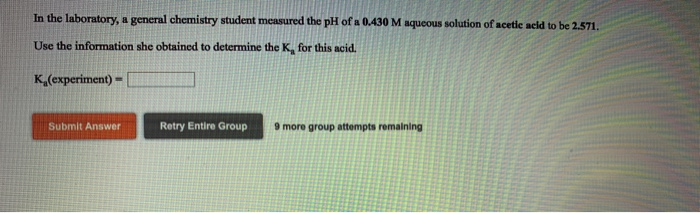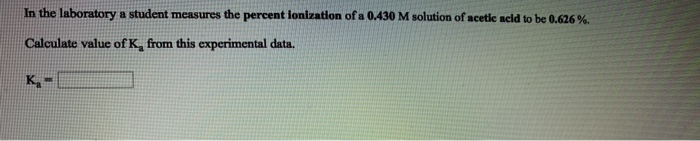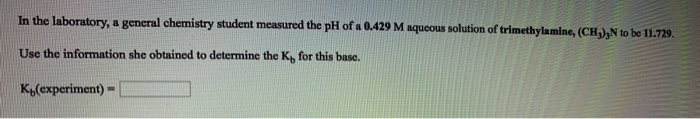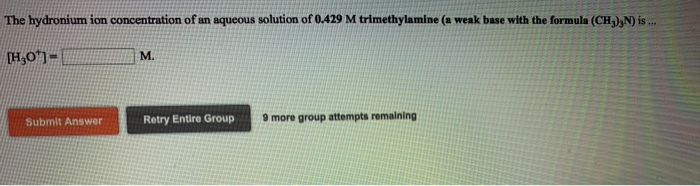In the laboratory, a general chemistry student measured the pH of a 0.430 M aqueous solution of acetie acid to be 2.571. Use the information she obtained to determine the K, for this acid. Ka(experiment)- Submit Answer Retry Entire Group 9 more group attempts remaining
In the laboratory a student measures the percent ionization of a 0.430 M solution of acetic acid to be 0626 %. Calculate value of Ka from this experimental data. Ka-l
In the laboratory, a general chemistry student measured the pH of a 0429 M aqueous solution of trimethylamine, (CH), to be 11729 Use the information she obtained to determine the Kp for this base. K,(experiment)-
We were unable to transcribe this image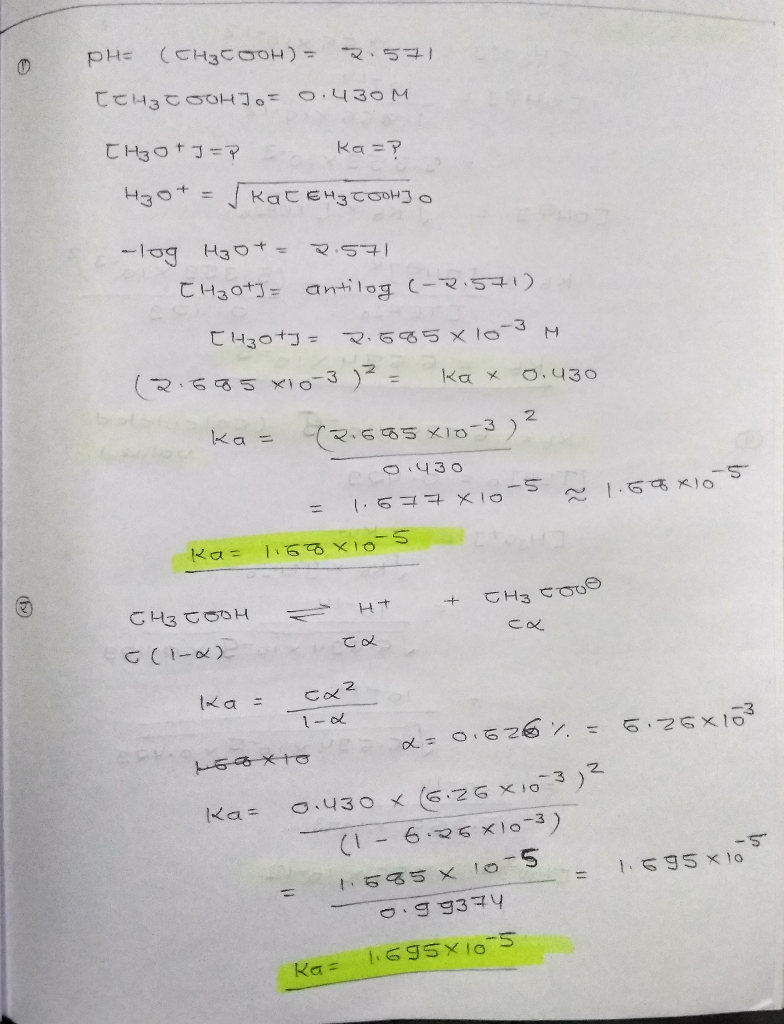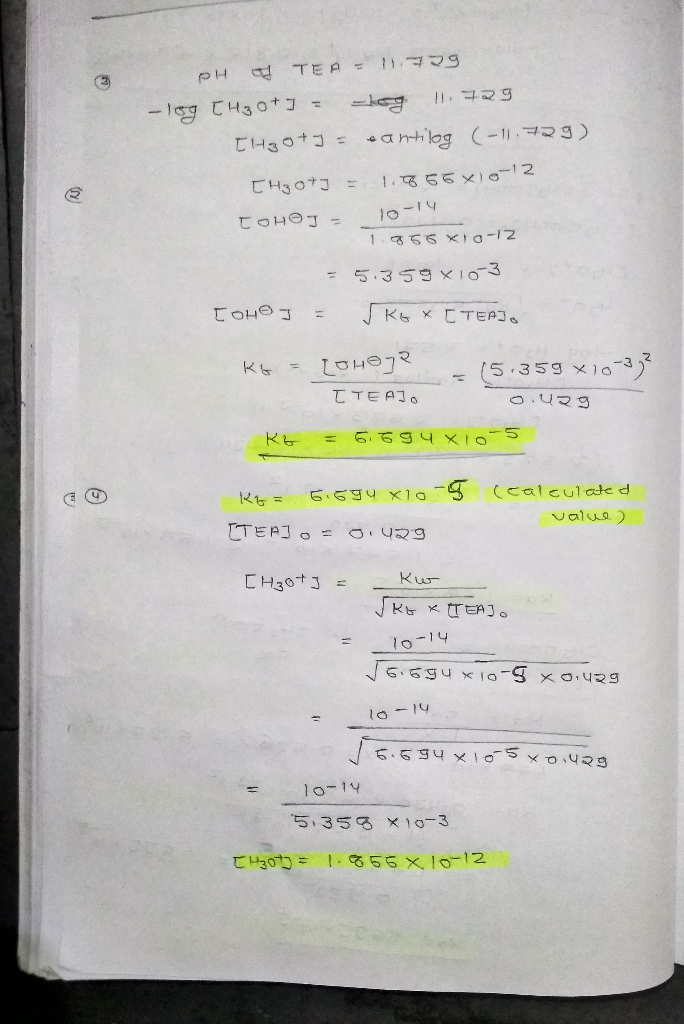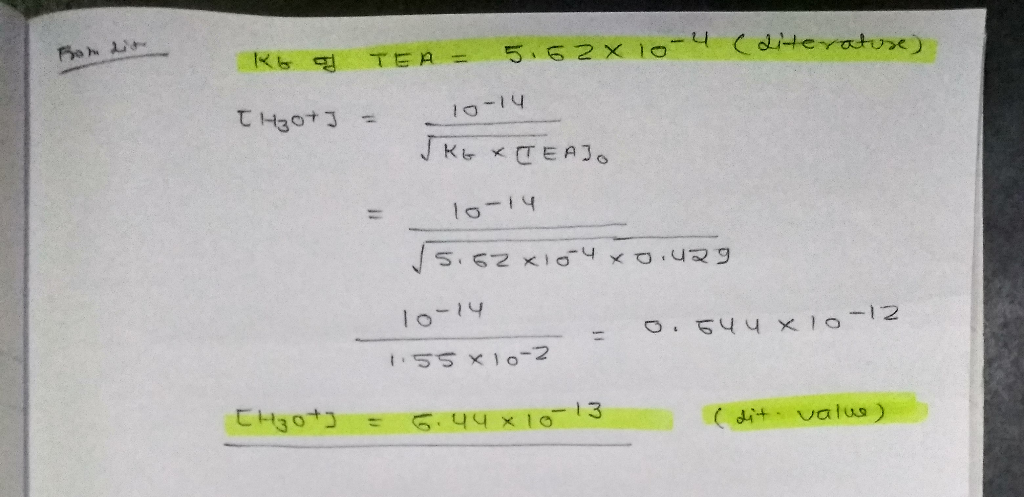#### Earn Coin

Coins can be redeemed for fabulous gifts.

Similar Homework Help Questions
• ### In the laboratory, a general chemistry student measured the pH of a 0.488 M aqueous solution...

In the laboratory, a general chemistry student measured the pH of a 0.488 M aqueous solution of hydrofluoric acid to be 1.711. Use the information she obtained to determine the Ka for this acid. Ka(experiment) = In the laboratory, a general chemistry student measured the pH of a 0.488 M aqueous solution of benzoic acid, C6H5COOH to be 2.273. Use the information she obtained to determine the Ka for this acid. Ka(experiment) = In the laboratory, a general chemistry student...

• ### In the laboratory, a general chemistry student measured the pH of a 0.534 M aqueous solution...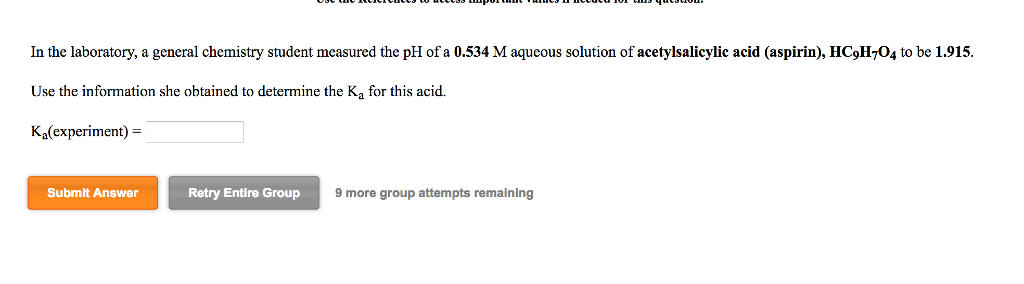In the laboratory, a general chemistry student measured the pH of a 0.534 M aqueous solution of acetylsalicylic acid (aspirin), HC9H704 to be 1.915. Use the information she obtained to determine the K, for this acid. Ka(experiment) = Submit Answer Retry Entire Group 9 more group attempts remaining

• ### 15.) a.)In the laboratory, a general chemistry student measured the pH of a 0.376 M aqueous...

15.) a.)In the laboratory, a general chemistry student measured the pH of a 0.376 M aqueous solution of nitrous acid to be 1.872. Use the information she obtained to determine the Ka for this acid. Ka(experiment) = b.)In the laboratory, a general chemistry student measured the pH of a 0.376 M aqueous solution of nitrous acid to be 1.870. Use the information she obtained to determine the Ka for this acid. Ka(experiment) = c.)In the laboratory, a general chemistry student...

• ### In the laboratory, a general chemistry student measured the pH of a 0.506 M aqueous solution...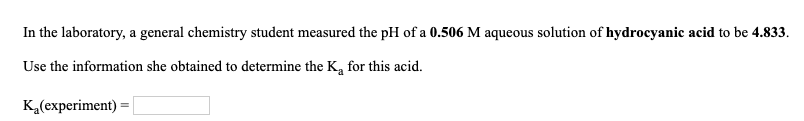In the laboratory, a general chemistry student measured the pH of a 0.506 M aqueous solution of hydrocyanic acid to be 4.833. Use the information she obtained to determine the K, for this acid. K (experiment) In the laboratory, a general chemistry student measured the pH of a 0.540 M aqueous solution of benzoic acid, CH3COOH to be 2.218. Use the information she obtained to determine the K, for this acid. K (experiment)

• ### In the laboratory, a general chemistry student measured the pH of a 0.392 M aqueous solution...

In the laboratory, a general chemistry student measured the pH of a 0.392 M aqueous solution of formic acid, HCOOH to be 2.059.   Use the information she obtained to determine the Ka for this acid.   Ka(experiment) =

• ### In the laboratory, a general chemistry student measured the pH of a 0.464 M aqueous solution...

In the laboratory, a general chemistry student measured the pH of a 0.464 M aqueous solution of formic acid, HCOOH to be 2.057.   Use the information she obtained to determine the Ka for this acid.   Ka(experiment) =

• ### In the laboratory, a general chemistry student measured the pH of a 0.329 M aqueous solution...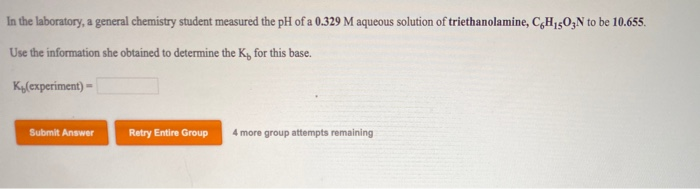In the laboratory, a general chemistry student measured the pH of a 0.329 M aqueous solution of triethanolamine, CHON to be 10.655. Use the information she obtained to determine the K for this base. Ky(experiment) - Submit Answer Retry Entire Group 4 more group attempts remaining

• ### In the laboratory, a general chemistry student measured the pH of a 0.370 M aqueous solution...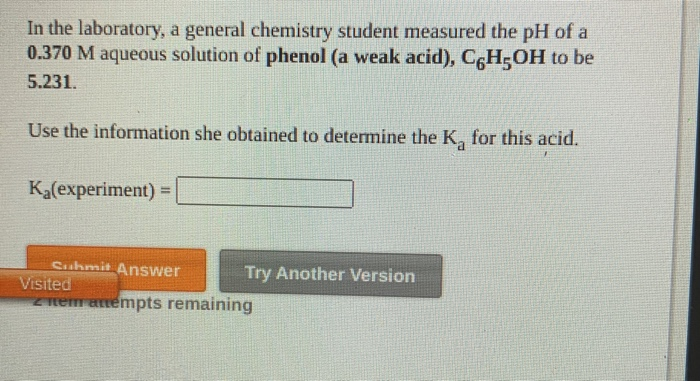In the laboratory, a general chemistry student measured the pH of a 0.370 M aqueous solution of phenol (a weak acid), C6H OH to be 5.231. Use the information she obtained to determine the K, for this acid. Ka(experiment) = Cushmit Answer Try Another Version Visited tremenempts remaining

• ### In the laboratory, a general chemistry student measured the pH of a 0.579 M aqueous solution...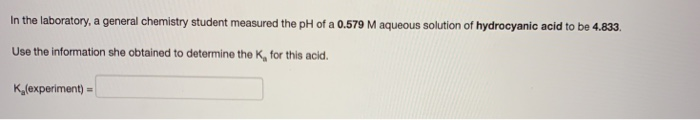In the laboratory, a general chemistry student measured the pH of a 0.579 M aqueous solution of hydrocyanic acid to be 4.833. Use the information she obtained to determine the K, for this acid. K(experiment)

• ### In the laboratory, a general chemistry student measured the pH of a 0.508 M aqueous solution...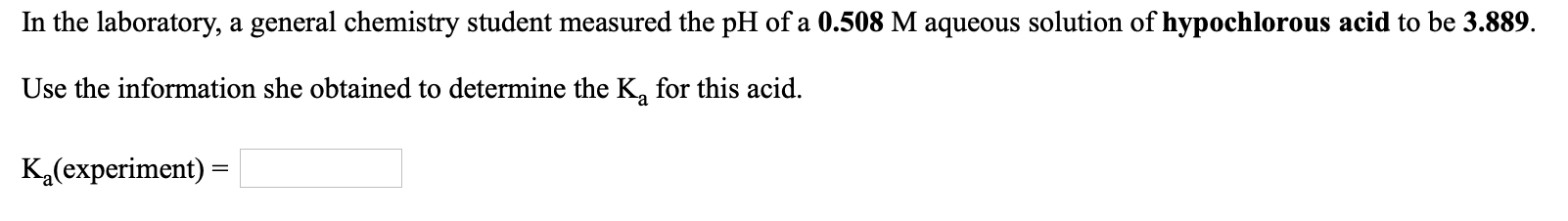In the laboratory, a general chemistry student measured the pH of a 0.508 M aqueous solution of hypochlorous acid to be 3.889. Use the information she obtained to determine the K, for this acid. K (experiment) =